# Approximating Real World Objects with Geometric Shapes

Instructor: Yuanxin (Amy) Yang Alcocer

Amy has a master's degree in secondary education and has taught math at a public charter high school.

In this lesson, you'll learn that geometric shapes can actually make your calculations easier. Instead of making lots of little calculations, you can use geometry to make just a few calculations to find what you need.

## A Real-World Object

Math is not just a topic you have to get through. No, it's everywhere in the real world. Just look at some of the buildings, bicycles, and cars around you: all were built with mathematics. Take a closer look, and you'll start to see that many of these objects look like geometric shapes.

A geometric shape is a shape that doesn't change when moved around. It's made up of points and lines. Basic examples include the triangle, rectangle, and circle. Your house might look like a triangle placed on top of a rectangle. A car's wheels are circles. So are your bicycle's wheels. A bus looks like rectangles on circles. You can use geometric shapes to approximate objects in the real world. It's an approximation because you're not accounting for every single curve in the real-world object. You can use these approximations to make your problem solving easier while still coming up with a usable answer.Now, let's look at what Dave the designer is up to. He uses math and geometry all the time in his work. Right now, he's designing a custom vase for a customer's upcoming wedding. The customer loves the number 5 and wants that number prominently incorporated into her wedding. So, she has asked Dave to design a pentagonal vase, a vase with 5 sides.

## Approximating with Geometric Shapes

Dave can use his awesome geometry skills to help him design the vase. See, a pentagonal vase is actually the geometric shape of a pentagon stacked one on top of the other to form a vase with a hollow interior. So, to figure out about how much material he needs, he can use geometry and its associated formulas to help him.

First, picture the pentagonal vase. Its base is that of a pentagon. The sides go up from there. It'll have five flat sides with each side being a rectangle.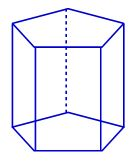With this shape, Dave can go ahead with his formulas. He needs enough material for the base of the vase as well as the sides of the vase, so he needs to figure out the area of the base and the sides. The formula for the area of a pentagon is this.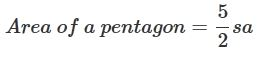The s stands for the side of the pentagon and the a stands for the apothem of the pentagon. Remember, the apothem is the distance from the center of a regular pentagon whose sides are all the same to the midpoint of each side.

To find the area of each rectangle, he'll need to use this formula.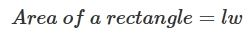The l stands for the length of the rectangle, and w stands for the width of the rectangle.

After making these calculations, Dave will need to add up the five sides and the base.

With a side measurement s of 7 inches for the pentagonal base and an apothem of 4.82 inches for the pentagon, the pentagon's area is calculated like this: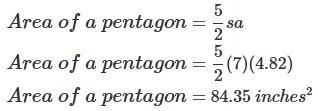With a height of 12 inches, the rectangles that make up the sides of the vase each measure 7 inches by 12 inches. The area of each of the sides of the vase is then calculated like this: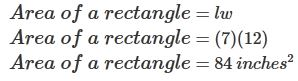To unlock this lesson you must be a Study.com Member.

### Register to view this lesson

Are you a student or a teacher?